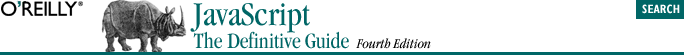home | O'Reilly's CD bookshelfs | FreeBSD | Linux | Cisco | Cisco Exam## 3.4. Functions

```function square(x)  // The function is named square. It expects one argument, x.
{                   // The body of the function begins here.
return x*x;       // The function squares its argument and returns that value.
}                   // The function ends here. ```
```y = Math.sin(x);
y = square(x);
d = compute_distance(x1, y1, z1, x2, y2, z2);
move( ); ```

Since functions are values just like numbers and strings, they can be assigned to object properties just like other values can. When a function is assigned to a property of an object (the object data type and object properties are described in Section 3.5), it is often referred to as a method of that object. Methods are an important part of object-oriented programming. We'll see more about them in Chapter 8.